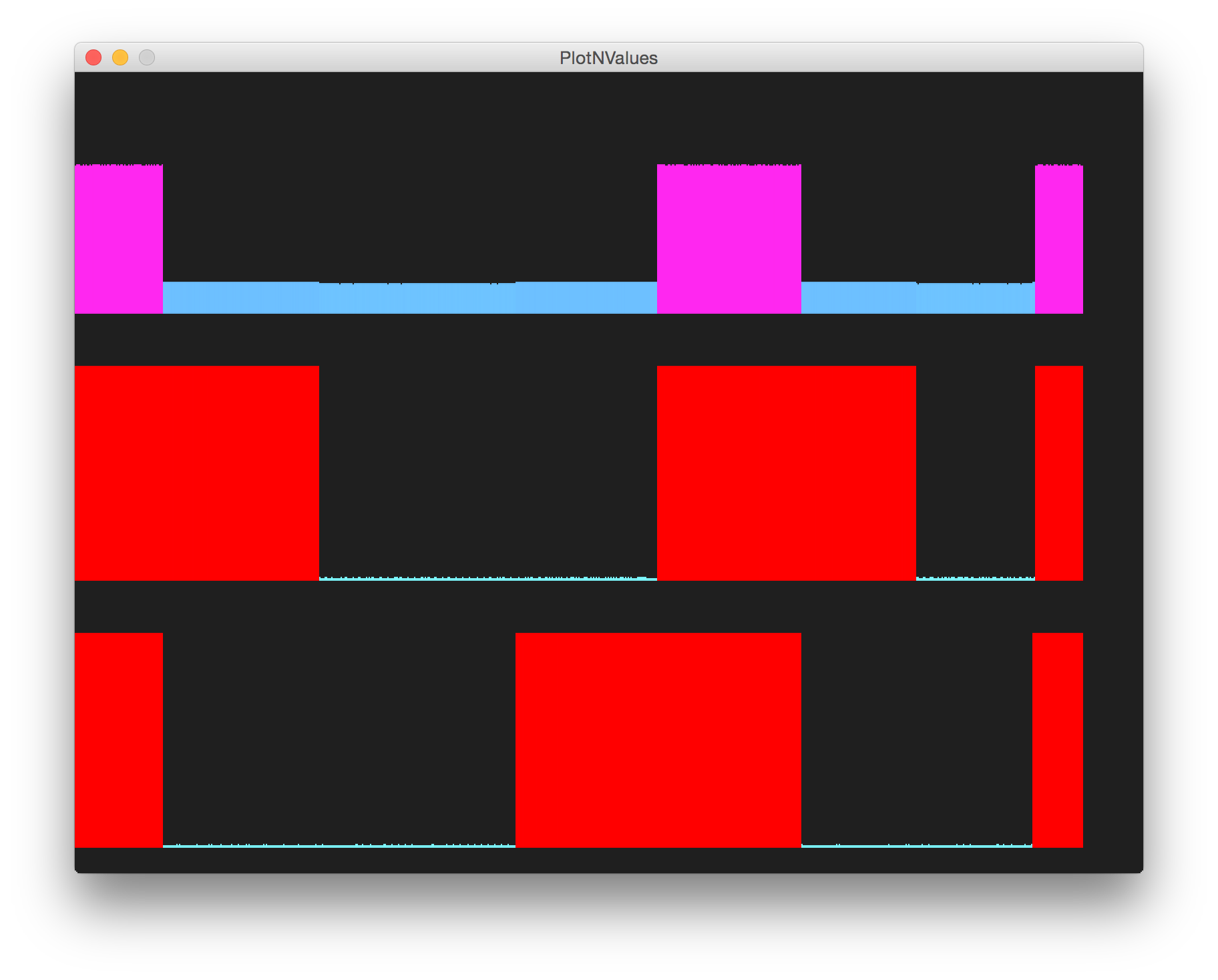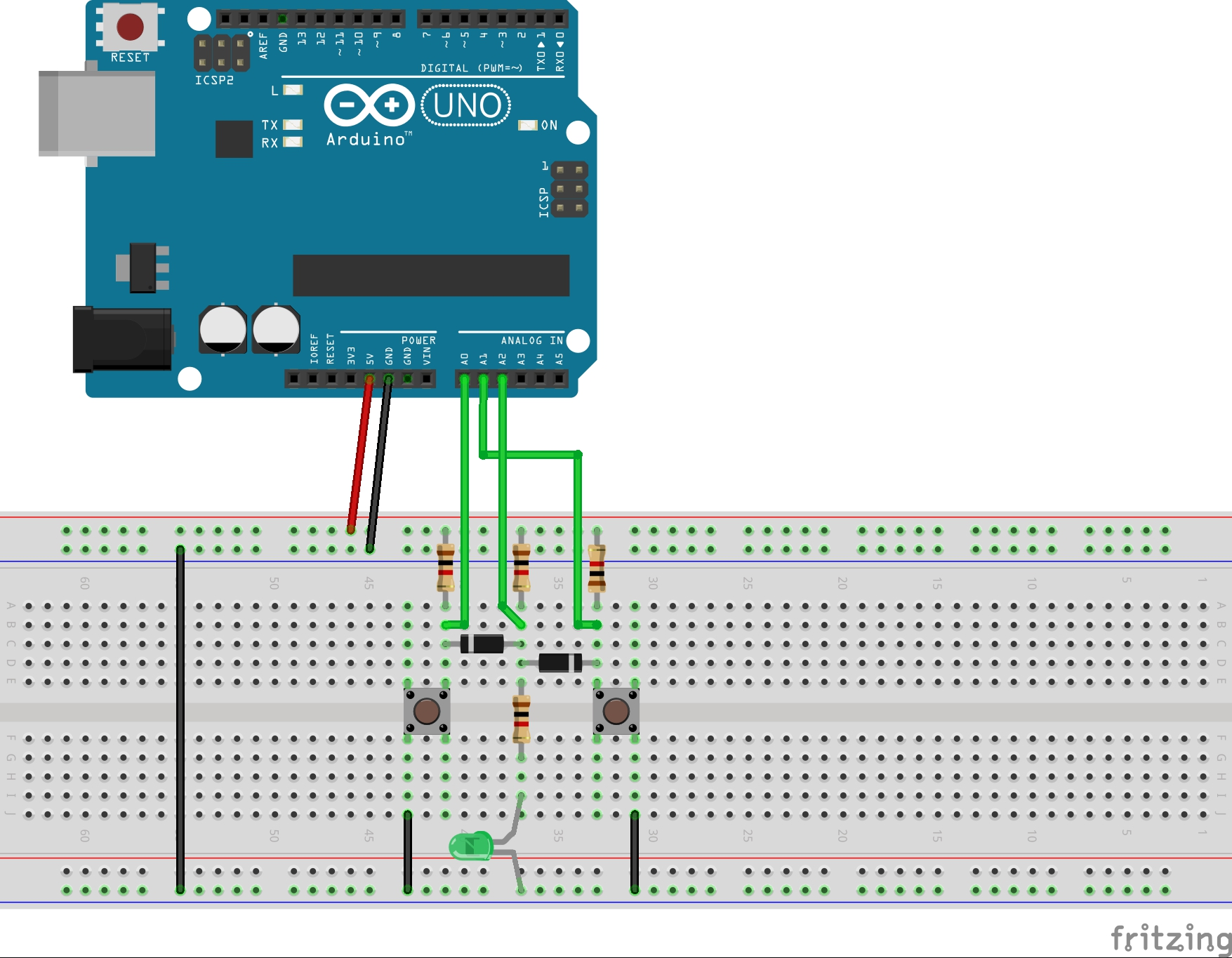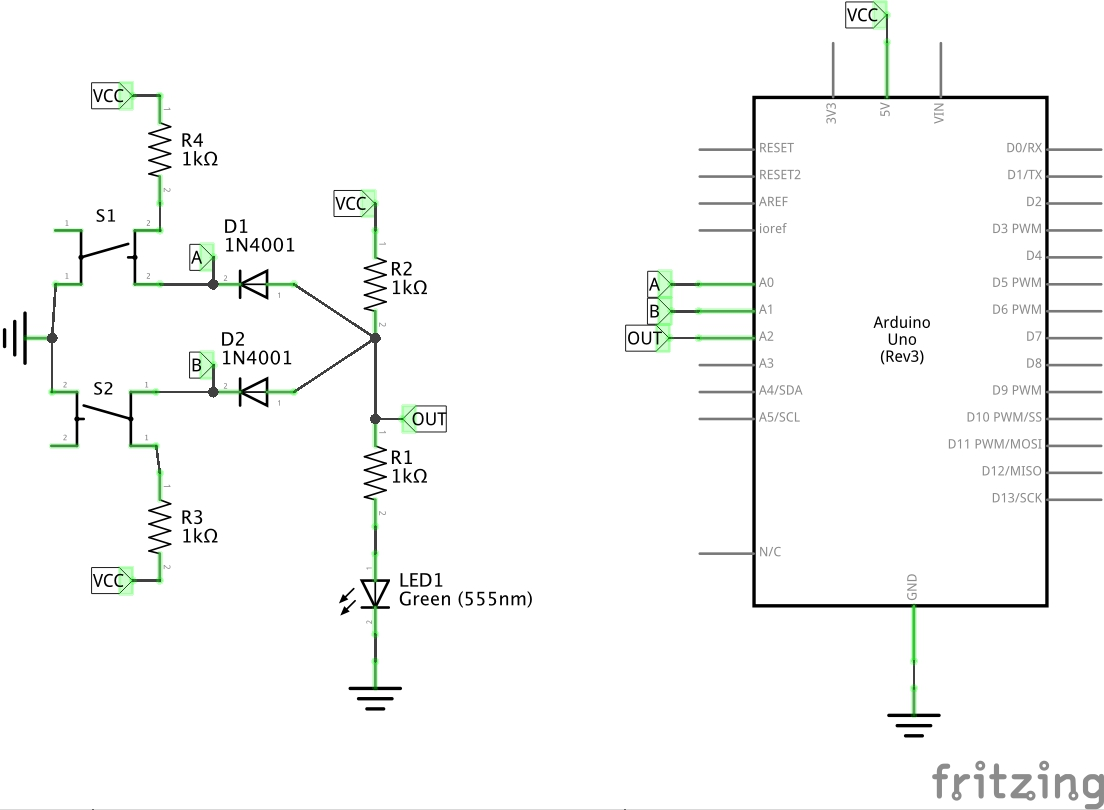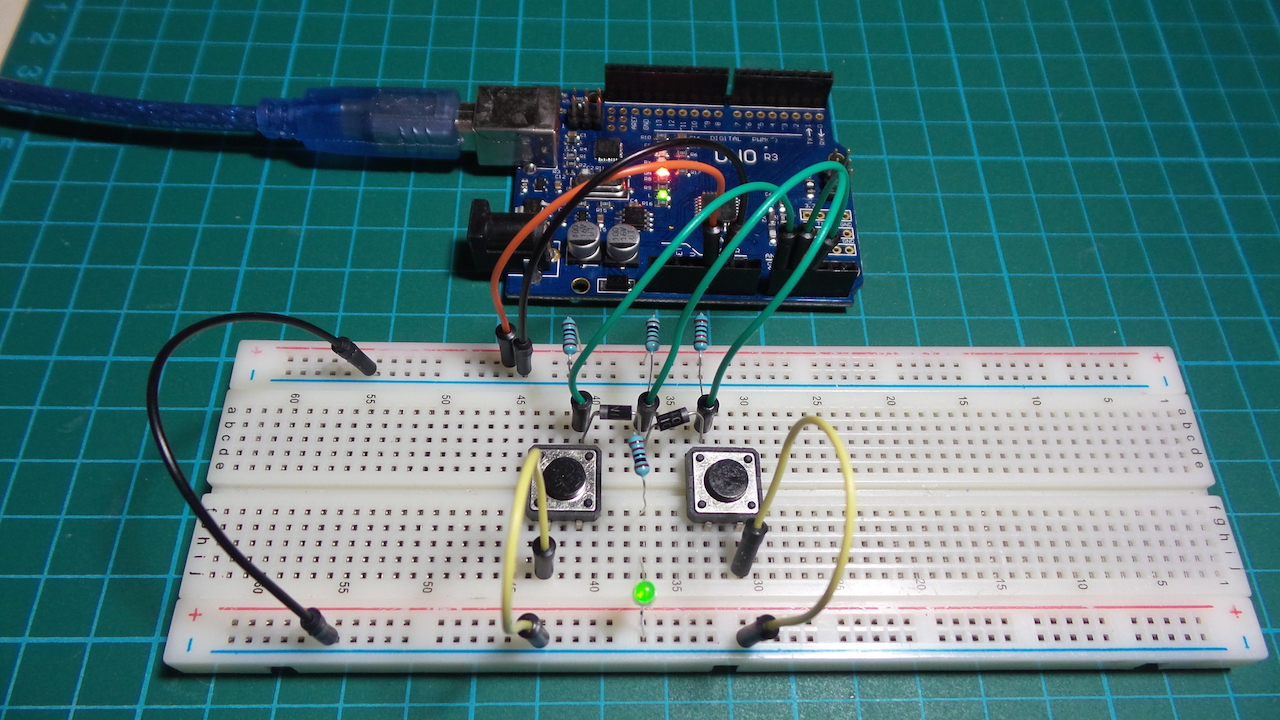# #110 DiodeLogic/AND

Test the basic diode-logic AND gate

Diode logic was used extensively in early computers but is largely obsolete now, since it cannot isolate inputs or outputs without additional circuitry, and there will be at least a 1-diode voltage drop to deal with across the gate.

It is interesting to see how various logic gate may be realised with diodes (and resistors) alone. Here is the basic AND gate.

Output is high only when all the inputs are high:

A B OUT
0 0 0
0 1 0
1 0 0
1 1 1

In this circuit with VCC of 5V and where Vf is the diode forward voltage of approx. 0.7V, Vr2 is the voltage drop across R2:

• Out logical “0” == Vf ~> 0.7V
• Out logical “1” == VCC - Vr2 ~> 3.4V

Note that with a normally-open switch, it engages logical “0” when closed, and logical “1” when open .. so the switch behaviour may appear inverse (more like a NOR gate) to the actual electrical behaviour. This apparently contradictory behaviour is resolved if a normally-closed switch is used.

The Arduino is not a core part of the circuit. It only does the following:

• provides +5V power supply (for convenience)
• measures the input and output voltages for plotting. It uses the PlotNValues sketch for this purpose.

Here’s a sample trace. The upper trace is the output, the lower traces the inputs.## Construction